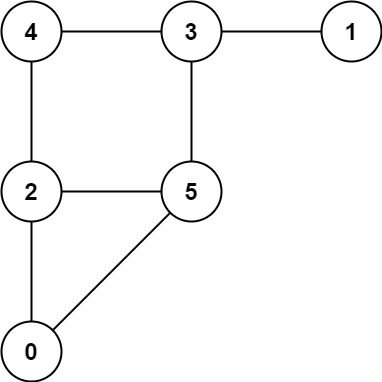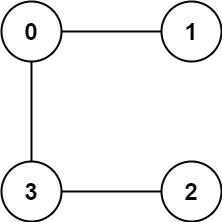Formatted question description: https://leetcode.ca/all/913.html

# 913. Cat and Mouse (Hard)

A game on an undirected graph is played by two players, Mouse and Cat, who alternate turns.

The graph is given as follows: graph[a] is a list of all nodes b such that ab is an edge of the graph.

The mouse starts at node 1 and goes first, the cat starts at node 2 and goes second, and there is a hole at node 0.

During each player's turn, they must travel along one edge of the graph that meets where they are.  For example, if the Mouse is at node 1, it must travel to any node in graph.

Additionally, it is not allowed for the Cat to travel to the Hole (node 0.)

Then, the game can end in three ways:

• If ever the Cat occupies the same node as the Mouse, the Cat wins.
• If ever the Mouse reaches the Hole, the Mouse wins.
• If ever a position is repeated (i.e., the players are in the same position as a previous turn, and it is the same player's turn to move), the game is a draw.

Given a graph, and assuming both players play optimally, return

• 1 if the mouse wins the game,
• 2 if the cat wins the game, or
• 0 if the game is a draw.

Example 1:Input: graph = [[2,5],,[0,4,5],[1,4,5],[2,3],[0,2,3]]
Output: 0


Example 2:Input: graph = [[1,3],,,[0,2]]
Output: 1


Constraints:

• 3 <= graph.length <= 50
• 1 <= graph[i].length < graph.length
• 0 <= graph[i][j] < graph.length
• graph[i][j] != i
• graph[i] is unique.
• The mouse and the cat can always move.

Related Topics:

Similar Questions:

## Solution 1.

// OJ: https://leetcode.com/problems/cat-and-mouse/
// Time: O(N^3)
// Space: O(N^2)
class Solution {
vector<array<int, 3>> parents(vector<vector<int>> &G, int m, int c, int t) {
vector<array<int, 3>> ans;
if (t == 2) {
for (int m2 : G[m]) ans.push_back({m2, c, 3 - t});
} else {
for (int c2 : G[c]) {
if (c2 > 0) ans.push_back({m, c2, 3 - t});
}
}
return ans;
}
public:
int catMouseGame(vector<vector<int>>& G) {
int N = G.size(), DRAW = 0, MOUSE = 1, CAT = 2;
int color = {}, degree = {};
for (int i = 0; i < N; ++i) {
for (int j = 0; j < N; ++j) {
degree[i][j] = G[i].size();
degree[i][j] = G[j].size();
for (int v : G[j]) {
if (v == 0) {
degree[i][j]--;
break;
}
}
}
}
queue<array<int, 4>> q;
for (int i = 0; i < N; ++i) {
for (int t = 1; t <= 2; ++t) {
color[i][t] = MOUSE;
q.push({0, i, t, MOUSE});
if (i > 0) {
color[i][i][t] = CAT;
q.push({i, i, t, CAT});
}
}
}
while (q.size()) {
auto [i, j, t, c] = q.front();
q.pop();
for (auto [i2, j2, t2] : parents(G, i, j, t)) {
if (color[i2][j2][t2] == DRAW) {
if (t2 == c) {
color[i2][j2][t2] = c;
q.push({i2, j2, t2, c});
} else if (--degree[i2][j2][t2] == 0) {
color[i2][j2][t2] = 3 - t2;
q.push({i2, j2, t2, 3 - t2});
}
}
}
}
return color;
}
};


Java

• class Solution {
public int catMouseGame(int[][] graph) {
int nodes = graph.length;
int[][][] memo = new int[nodes * 2][nodes][nodes];
for (int i = nodes * 2 - 1; i >= 0; i--) {
for (int j = nodes - 1; j >= 0; j--) {
for (int k = nodes - 1; k >= 0; k--)
memo[i][j][k] = -1;
}
}
return catMouseGame(graph, memo, 0, 1, 2);
}

public int catMouseGame(int[][] graph, int[][][] memo, int step, int mouse, int cat) {
int nodes = graph.length;
if (step == nodes * 2)
return 0;
else if (memo[step][mouse][cat] != -1)
return memo[step][mouse][cat];
else if (mouse == cat) {
memo[step][mouse][cat] = 2;
return 2;
} else if (mouse == 0) {
memo[step][mouse][cat] = 1;
return 1;
} else {
if (step % 2 == 0) {
boolean catWin = true;
int[] nextMouseNodes = graph[mouse];
for (int nextMouse : nextMouseNodes) {
int nextResult = catMouseGame(graph, memo, step + 1, nextMouse, cat);
if (nextResult == 1) {
memo[step][mouse][cat] = 1;
return 1;
} else if (nextResult == 0)
catWin = false;
}
if (catWin)
memo[step][mouse][cat] = 2;
else
memo[step][mouse][cat] = 0;
} else {
boolean mouseWin = true;
int[] nextCatNodes = graph[cat];
for (int nextCat : nextCatNodes) {
if (nextCat != 0) {
int nextResult = catMouseGame(graph, memo, step + 1, mouse, nextCat);
if (nextResult == 2) {
memo[step][mouse][cat] = 2;
return 2;
} else if (nextResult == 0)
mouseWin = false;
}
}
if (mouseWin)
memo[step][mouse][cat] = 1;
else
memo[step][mouse][cat] = 0;
}
return memo[step][mouse][cat];
}
}
}

• // OJ: https://leetcode.com/problems/cat-and-mouse/
// Time: O(N^3)
// Space: O(N^2)
class Solution {
vector<array<int, 3>> parents(vector<vector<int>> &G, int m, int c, int t) {
vector<array<int, 3>> ans;
if (t == 2) {
for (int m2 : G[m]) ans.push_back({m2, c, 3 - t});
} else {
for (int c2 : G[c]) {
if (c2 > 0) ans.push_back({m, c2, 3 - t});
}
}
return ans;
}
public:
int catMouseGame(vector<vector<int>>& G) {
int N = G.size(), DRAW = 0, MOUSE = 1, CAT = 2;
int color = {}, degree = {};
for (int i = 0; i < N; ++i) {
for (int j = 0; j < N; ++j) {
degree[i][j] = G[i].size();
degree[i][j] = G[j].size();
for (int v : G[j]) {
if (v == 0) {
degree[i][j]--;
break;
}
}
}
}
queue<array<int, 4>> q;
for (int i = 0; i < N; ++i) {
for (int t = 1; t <= 2; ++t) {
color[i][t] = MOUSE;
q.push({0, i, t, MOUSE});
if (i > 0) {
color[i][i][t] = CAT;
q.push({i, i, t, CAT});
}
}
}
while (q.size()) {
auto [i, j, t, c] = q.front();
q.pop();
for (auto [i2, j2, t2] : parents(G, i, j, t)) {
if (color[i2][j2][t2] == DRAW) {
if (t2 == c) {
color[i2][j2][t2] = c;
q.push({i2, j2, t2, c});
} else if (--degree[i2][j2][t2] == 0) {
color[i2][j2][t2] = 3 - t2;
q.push({i2, j2, t2, 3 - t2});
}
}
}
}
return color;
}
};

• class Solution(object):
def catMouseGame(self, graph):
"""
:type graph: List[List[int]]
:rtype: int
"""
N = len(graph)
MOUSE, CAT = 1, 2
# mouse, cat, turn
color = [[ * 3 for i in range(N)] for j in range(N)]
q = collections.deque()
for i in range(1, N):
for t in range(1, 3):
color[i][t] = 1
q.append((0, i, t))
color[i][i][t] = 2
q.append((i, i, t))
while q:
curStatus = q.popleft()
cat, mouse, turn = curStatus
for preStatus in self.findAllPrevStatus(graph, curStatus):
preCat, preMouse, preTurn = preStatus
if color[preCat][preMouse][preTurn] != 0:
continue
if color[cat][mouse][turn] == 3 - turn:
color[preCat][preMouse][preTurn] = preTurn
q.append(preStatus)
elif self.allNeighboursWin(color, graph, preStatus):
color[preCat][preMouse][preTurn] = 3 - preTurn
q.append(preStatus)
return color

def findAllPrevStatus(self, graph, curStatus):
ret = []
mouse, cat, turn = curStatus
if turn == 1:
for preCat in graph[cat]:
if preCat == 0:
continue
ret.append((mouse, preCat, 2))
else:
for preMouse in graph[mouse]:
ret.append((preMouse, cat, 1))
return ret

def allNeighboursWin(self, color, graph, status):
mouse, cat, turn = status
if turn == 1:
for nextMouse in graph[mouse]:
if color[nextMouse][cat] != 2:
return False
elif turn == 2:
for nextCat in graph[cat]:
if nextCat == 0:
continue
if color[mouse][nextCat] != 1:
return False
return True